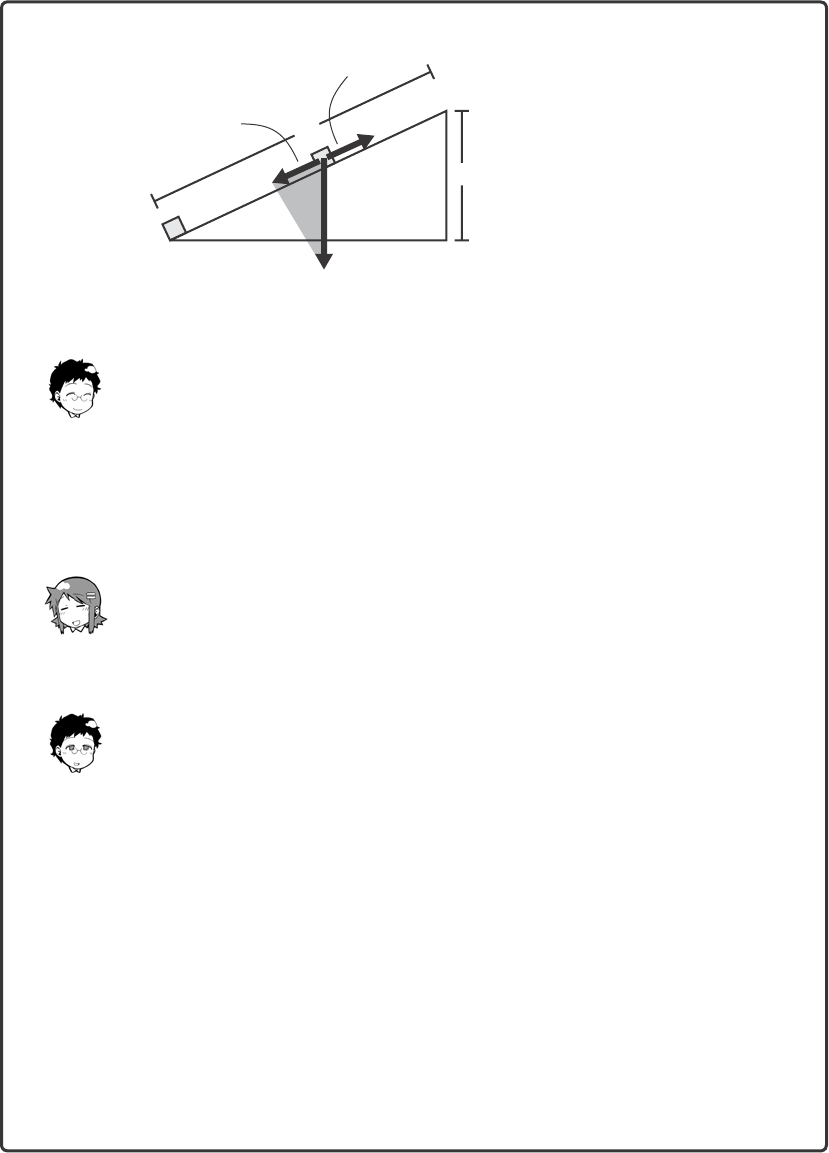172 Chapter 4 Energy
Laboratory
Work and the Conservation of Energy
Let’s consider a scenario in which we are lifting a heavy load to a certain
height. The simplest way to do this is to lift straight up. The following dia-
gram shows how it looks.
We are lifting a load with mass m to height h.
Let’s consider how much work we must do to lift the load to a height of h
by imposing a force equal to the force of gravity of the mass—that is, we’ll
impose a force upward equivalent to the force downward from gravity.
Assuming g for gravitational acceleration, we know that the force down-
ward is mg:
work upward = force of lifting × height h = mgh
Note that for simplicity’s sake, we won’t take into account friction or air
resistance in these examples. But this is a hard way to lift something so
heavy!
Hmm . . . maybe it’d be easier if we pushed the load up a ramp.
Yes, let’s consider the case of pushing the load up an incline.
Lifting force
h
mgWork and the Conservation of Energy 173
Look at this diagram. The magnitude of the force needed to push the load
up this ramp (F) is equal to the component of the force of gravity parallel
to the ramp (PR). So, if the ramp has a length of d, the work required to
move the load to height h can be represented as:
work = Fd
Now, you know intuitively that F is smaller than mg, and d is larger than h.
That makes sense. Is that why it takes the same amount of work to push
the load up a ramp as it does to lift the load straight up?
Yes, indeed. Now let’s show why this works, mathematically. r ABC repre-
sents the ramp in the figure, and rPQR represents the composition of the
force mg. These two triangles are similar—this means that CAB = RPQ.
This also means that the proportion of their corresponding sides must be
the same, as well. Thus, the following must be true:
AB
AC
=
PQ
PR
Let’s make this a little less abstract. The line segment AB equals d (length
of ramp) and AC equals h (height). Similarly, the line segment PQ equals
mg (the force downward, due to gravity), while PR equals F (the force
applied to offset a portion of that force).
Lifting
force (F)
A
CB
R
P
Q
Component of
weight (PR)
d
Weight (mg)
h174 Chapter 4 Energy
That means:
d
h
=
mg
F
Look, with just a little rearranging of this equation we get the following:
Fd = mgh
Therefore, the work to lift a load using a ramp must be equal to the work
to lift that load straight upward.
Also, please note that our results are the same, regardless of the angle
of the ramp. Given the conservation of energy, regardless of the lifting
route, the work done for lifting an object with mass m to height h is equal
to the following:
force required to balance gravity × height = mgh
So, whatever method you use to lift something, the amount of work you
do is the same.
To put it another way, your work increases the potential energy of the load
by mgh.
And I bet it works for negative work, too. That is, you’d see a decrease in
potential energy of mgh if you lower an object by mgh.
Yep, that’s right.Work and Energy
Work isn’t
onlydone when
increasing or
decreasing
potential
energy.
Work can
alsoaect the
kinetic energy
ofan object!
You mean work is
also done when
we move an object
or bring a moving
object to a halt?
Are you sti
listening to me,
Ninomiya-san?
Yes, go on
with the
leon.
We.
Ahem.
While you impose a
force for a given
distance on an object
at rest, that object’s
kinetic energy
increases.
Imposing a
force on an
object
Generates
kinetic energy.
What’s
haening?
I fl
like I’m
shrinking...
I’m a
grown
up!
Aw!
How
cute!
What the...?
F
SqualWhoa. This is true
forobjects in
motion as we. In
other words, the
kinetic energy of
an object increases
even more
if you impose
a force in the
direction of the
object’s motion.
For some
reason, you
remind me of a
pachinko ba.
Since energy is
conserved, we know
thefoowing:
work done on the object =
change in the object’s kinetic energy
This relationship
must hold true.
Ah, yes.
If the force we
impose on an
object is in the
direction of the
object’s
motion—
that is, when the
force and velocity
are parael—we
wi do positive
work.
176 Chapter 4 Energy

Get The Manga Guide to Physics now with the O’Reilly learning platform.

O’Reilly members experience books, live events, courses curated by job role, and more from O’Reilly and nearly 200 top publishers.